# GSEB Solutions Class 8 Maths Chapter 13 Direct and Inverse Proportions Ex 13.2

Gujarat Board GSEB Textbook Solutions Class 8 Maths Chapter 13 Direct and Inverse Proportions Ex 13.2 Textbook Questions and Answers.

## Gujarat Board Textbook Solutions Class 8 Maths Chapter 13 Direct and Inverse Proportions Ex 13.2

Question 1.
Which of the following are in inverse proportion?

1. The number of workers on a job and the time to complete the job.
2. The time taken for a journey and the distance travelled in a uniform speed.
3. Area of cultivated land and the crop harvested.
4. The time taken for a fixed journey and the speed of the vehicle.
5. The population of a country and the area of land per person.

Solution:
REMEMBER If an increase in one quantity brings about a corresponding decrease in the other and vice versa, then the two quantities vary inversely.

1. If number of workers are increased then time to complete the job would decrease. It is a case of inverse variation.
2. For longer distance, more time would be required. It is not a case of inverse variation
3. For more area of land, more crops would be harvested. It is not a case of inverse variation.
4. If speed is more, time to cover a fixed distance would be less. It is a case of inverse variation.
5. For more population, less area per person would be required. It is a case of inverse variation.Question 2.
In a television game show, the prize money of ₹ 1,00,000 is to be divided equally amongst the winners. Complete the following table and find whether the prize money given to an individual winner is directly or inversely proportional to the number of winners?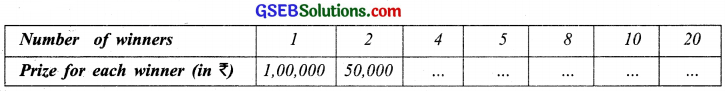Solution:
If more the number of winners, less is the prize money.
∴ It is a case of inverse proportion.Question 3.
Rehman is making a wheel using spokes. He wants to fix equal spokes in such a way that the angles between any pair of consecutive spokes are equal. Help him by completing the following table?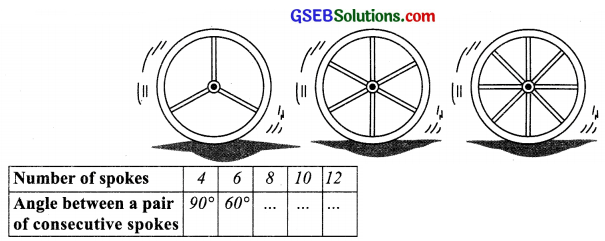1. Are the number of spokes and the angles formed between the pairs of consecutive spokes in in verse proportion?
2. Calculate the angle between a pair of consecutive spokes on a wheel with 15 spokes?
3. How many spokes would be needed, if the angle between a pair of consecutive spokes is 400?

Solution:
1. Obviously, more the number of spokes, less is the measure of angle between a pair of consecutive spokes.
∴ It is a case of inverse variation.
Thus, 4 × 90 = 8 × x1 ⇒ x1 = $$\frac{4×90}{8}$$ 45°
4 × 90 = 10 × x2 ⇒ x2 = $$\frac{4×90}{10}$$ 36°
4 × 90 = 12 × x3 ⇒ x3 = $$\frac{4×90}{12}$$ 30°
Thus, the table is completed as under2. Let the required measure of angle be x°.
∴ 15 × x° = 4 × 90° ⇒ x° = $$\frac{4×90}{15}$$ = 24°

3. Let required number of spokes be n.
∴ n × 40° = 40 × 90°
or n = $$\frac{4 \times 90^{\circ}}{40^{\circ}}$$ = 9
∴ The required number of spokes = 9Question 4.
If a box of sweets is divided among 24 children, they will get 5 sweets each. How many would each get, if the number of the children is reduced by 4?
Solution:
Reduced number of children = 24 – 4 = 20
Since, more the number of children, less is the quantity of sweets.
∴ It is a case of inverse variation.
i.e., 24 × 5 = 20 × x
or x = $$\frac{24×5}{20}$$ = 6
∴ Each student will get 6 sweets.

Question 5.
A farmer has enough food to feed 20 animals in his cattle for 6 days. How long would the food last f there were 10 more animal in his cattle?
Solution:
Number of animals added = 10
∴ Now, the total number of animals
= 10 + 20 = 30
For more number of animals, the food will last less number of days.
∴ It is a case of inverse variation.
Thus, we have
30 × x = 20 × 6
or x = $$\frac{20×6}{30}$$ = 4
Therefore, the food will now last for 4 days.Question 6.
A contractor estimates that 3 persons could rewire Jasminder’s house in 4 days. If he uses 4 persons instead of three, how long should they take to complete the job?
Solution:
More is the number of persons, less is the time to Complete the job.
∴ It is a case of inverse variation.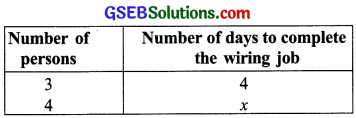∴ 3 × 4 = 4 × x or x = $$\frac{3×4}{4}$$ = 3
∴ The required number od days = 3

Question 7.
A batch of bottles were packed in 25 boxes with 12 bottles in each box. If the same batch is packed using 20 bottles in each box, how many boxes would be filled?
Solution:
Let the number of boxes required = x
We have:Since, more the number of bottles in a box, lesser will be the number of boxes required to be filled.∴ It is a case of inverse variation.
i.e., 12 × 25 = 20 × x
or x = $$\frac{12×25}{20}$$ = 15
Thus, the required number of boxes = 15Question 8.
A factory requires 42 machines to produce a given number of articles in 63 days. How many machines would be required to produce the same number of articles in 54 days?
Solution:
Let the number of machines required be x.
We have:∴ 42 × 63 = x × 54
or x = $$\frac{42×63}{54}$$ = 7 × 7 = 49
Thus, the required number of machines = 49

Question 9.
A car takes 2 hours to reach a destination by travelling at the speed of 60 km/h. How long will it take when the car travels at the speed of 80 km/h?
Solution:
More the speed, lesser the number of hours to travel a fixed distance.
∴ It is a case of inverse variations.
Now,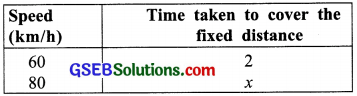∴ 60 × 2 = 80 × x
or x = $$\frac{60×2}{80}$$ = $$\frac{3}{2}$$ or 1$$\frac{1}{2}$$ hours
Thus the required number of hours = 1$$\frac{1}{2}$$Question 10.
Two persons could fit new window in a house in 3 days.

1. One of the persons fell ill before the work started. How long would the job take now?
2. How many persons would be needed to fit the windows in one day?

Solution:
1. Let the time taken by the remaining persons to complete the job be x.
∵ 2 persons – 1 person = 1 person and lesser the number of person, more will be the number of days to complete the job.
∴ It is a case of inverse proportion.
We have: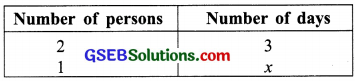∴ 2 × 3 = 1 × x
or x = $$\frac{2×3}{1}$$ = 6
∴ 1 person will complete the job in 6 days.

2. we have∴ 2 × 3 = 1 × x or x = $$\frac{2×3}{1}$$ = 6
∴ 6 persons will be required to complete the job in 1 day.Question 11.
A school has 8 periods a day each of 45 minutes duration. How long would each period be, if the school has 9 periods a day, assuming the number of school houres to be the same?
Solution:
For 9 periods, let the duration per period be x minutes.
∴ We have: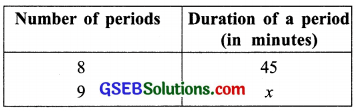For a fixed duration, more the periods, lesser will be the duration of one period.
∴ It is a case of inverse proportion.
i.e., 8 × 45 = 9 × x
or x = $$\frac{8×45}{9}$$ = 40
Thus, the required duration per period = 40 minutes.